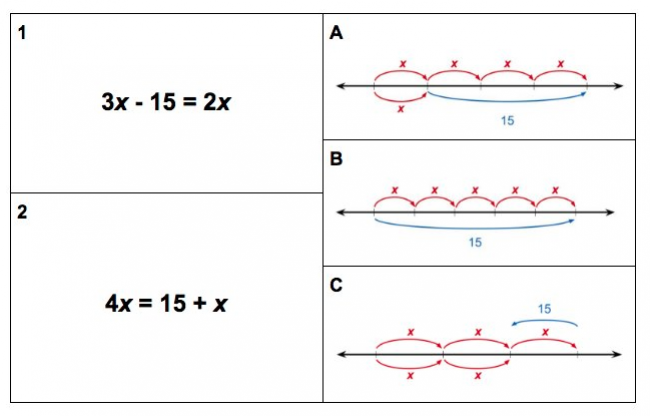# Equations on Number Lines

Preview:This task helps students see that quantities in equations can be represented as distances on a number line and that the equality of either side of the equation is represented by equivalent distances on the number line.

Grade:
Grade 9
Task Type:
Connecting Representations
Big Idea:
Grade 9: The principles and processes underlying operations with numbers apply equally to algebraic situations and can be described and analyzed.
Content:
includes distribution, variables on both sides of the equation, and collecting like terms, includes rational coefficients, constants, and solutions, solving and verifying 1 + 2x = 3 – 2/3(x + 6), solving symbolically and pictorially
Curricular Competency:
Reasoning and analyzing: Develop mental math strategies and abilities to make sense of quantities
Understanding and solving: Develop, demonstrate, and apply mathematical understanding through play, inquiry, and problem solving
Understanding and solving: Visualize to explore mathematical concepts
Understanding and solving: Develop and use multiple strategies to engage in problem solving
Communicating and representing: Communicate mathematical thinking in many ways
Communicating and representing: Use mathematical vocabulary and language to contribute to mathematical discussions
Communicating and representing: Explain and justify mathematical ideas and decisions
Communicating and representing: Represent mathematical ideas in concrete, pictorial, and symbolic forms
Connecting and reflecting: Reflect on mathematical thinking

## Add new comment

### Filtered HTML

• Web page addresses and e-mail addresses turn into links automatically.
• Allowed HTML tags: <a> <em> <strong> <cite> <blockquote> <code> <ul> <ol> <li>
• Lines and paragraphs break automatically.

### Plain text

• No HTML tags allowed.
• Web page addresses and e-mail addresses turn into links automatically.
CAPTCHA
This question is for testing whether or not you are a human visitor and to prevent automated spam submissions.
rrheobxshwquots